## "Live as if you were going to die tomorrow. Learn as if you were going to live forever" Mahatma Gandhi## Methods for estimating settlements from the spt

The SPT (Standard Penetration Test) is one of the most widely used field tests around the world, and is particularly useful for estimating short-term settlements in granular soils, in which it is practically impossible to obtain undisturbed samples for laboratory tests to determine their stress-strain behavior. Do you want to learn more? Continue reading...

### The SPT

The SPT (Standard Penetration Test) is a field test that is performed in a borehole, and basically consists of recording the number of blows required to drive a 2" diameter split-spoon type sampler, 30 cm into the ground, by means of the free fall of a 140 lb hammer, from a height of 76 cm. Of course, this test is standardized, and the most widely used standards that govern it are ISO-22476-3 and ASTM-D-1586 specifications. Figure 1 shows a simple schematic of the test.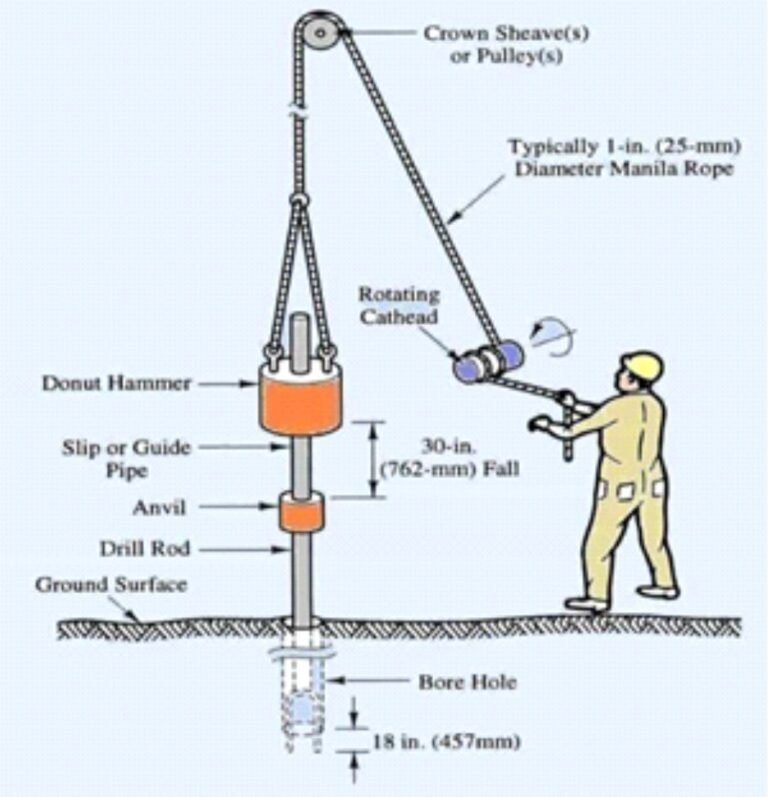Figure 1 The SPT

The test can be performed using all types of equipment (from portable machines to modern fully automated equipment), being very important to carry out the correction of the field NSPT value considering, for this application, the efficiency of the hammer by means of the CE factor.

Typically, the ratio of energy transmitted to the bars (ERr) is of the order of 50% to 60% of the maximum theoretical value of 475 J, although in practice this value can vary from 30% to 90%, depending on the driller and the equipment used to perform the SPT test (Kulhawy & Mayne, 1990). According to the above, it is clear the need to correct the NSPT value to consider the energy transmitted to the bars.

According to Amundaray (2006), it is recommended to consider CE = 0.75 (ERr = 45%) for conditions of little control in the field and CE = 1.0 (ERr = 60%) when good quality equipment and controlled sampling procedures are used, for drills equipped with Donut hammers. Seed et al (2003), recommend considering CE = 1.33 (ERr = 80%) for modern equipment with automatic hammers.

### Methodologies for estimating settlements from the SPT

Over the years, several methodologies have been developed to estimate settlement using the SPT. We will review here the most commonly used in practice in routine geotechnical studies.

Terzagui & Peck (1948) proposed a correlation based on the value of N60 to estimate the bearing capacity of a shallow foundation, for a settlement of 25 mm. This correlation, generalized for any value of settlement Se (in mm), resulted in the following expression: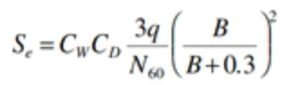Where: q = the contact pressure (in kPa), B = the width of the foundation (in m); CW = correction factor for the presence of the water table (considered equal to 1 if the water table is below a depth equal to 2B from the bearing depth, and equal to 2 if it is above that depth); and CD = the correction for the depth of embedment (equal to 1 - Df/4B, where Df is the depth of embedment).

Meyerhoff (1956, 1965) proposed the following expressions to estimate the settlement of a foundation, based on laboratory-analyzed models and field data of settlement of structures: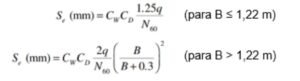The values of the CW and CD factors are determined considering the same criteria proposed by Terzaghi and Peck, mentioned above.

The last method to be reviewed here is the proposed by Peck & Bazaraa (1969), which is nothing more than a revision of the method initially proposed by Terzaghi & Peck in 1948. The expression they propose is the following: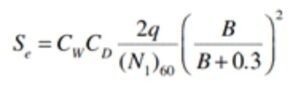Where: Se = settlement (in mm); q = contact pressure (in kPa); B = foundation width (in m); CW = correction factor for the presence of the water table; CD = correction for the depth of embedment; and (N1)60 = NSPT value corrected for energy and confinement.

The correction factor CW takes into account the influence of the water table, and it is obtained from the following expression:In this equation, σo = total overburden stress, and σ'o = effective overburden stress, both estimated at a depth equal to 0.5B below the foundation.

The overburden correction CD can be estimated with the following equation, where γ is the unit weight of the soil (in kN/m3):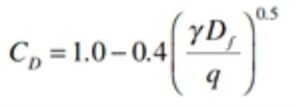Finally, the NSPT correction considering the confinement (for the correction for energy and obtaining N60, as we see in section 1 of this post), is made based on the following expressions: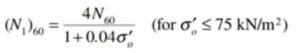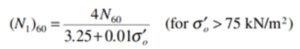As you can see, this method considers the effective overburden stress and the NSPT value corrected for overburden and drilling energy. Including these parameters, the settlements estimated in coarse soils from NSPT values are relatively close to the settlements observed in the field.

### Depth of influence and selection of the NSPT value to be used

A very important aspect to take into account when applying these methods, is the depth of influence of the foundation, since this depth is directly linked to the NSPT values to be used in the settlement estimations.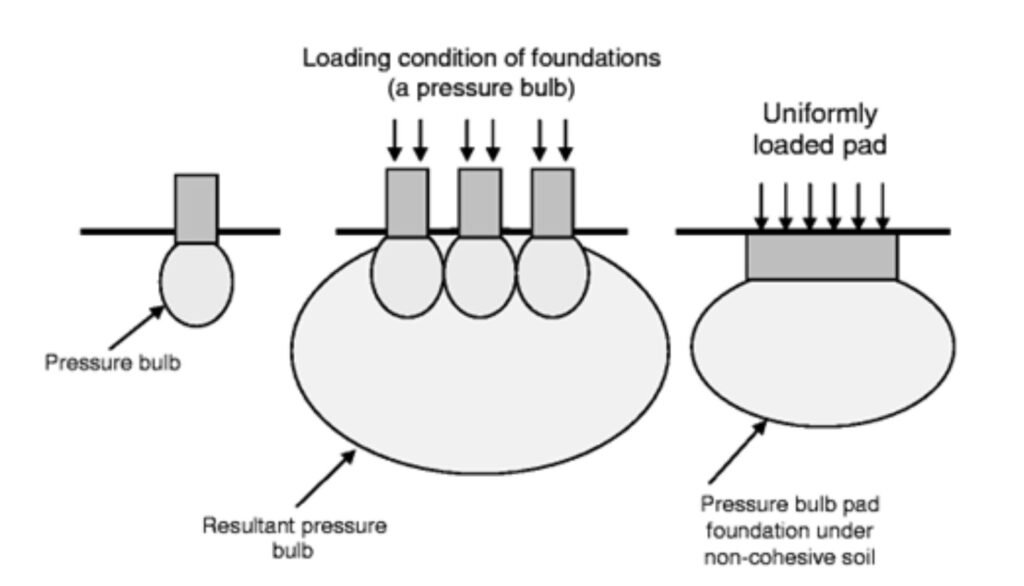Figure 2 Depth of influence below foundations (Source: modified from Zeitoun & Wakshal, 2012).

In general, the depth of influence for settlement estimation can be considered theoretically equal to 2B below shallow footing, and 4B for spread footings and slabs, where B is the width of the foundation. Thus, the value of N60 - or (N1)60, if the expression proposed by Peck & Bazaraa is used - to be considered in the estimates, must be equal to the average of the values of N60 - or (N1)60 - between the embedment depth and a depth equal to 2B or 4B (as applicable) below the foundation.

When will the depth of influence not be equal to 4B? Well, when, due to particularities of the subsoil, a significant increase of NSPT values is observed in the theoretical zone of influence. This would be evidence of the presence of a layer of high stiffness, located below a less stiff stratum, susceptible to deformation. If this is the case, the NSPT values of the less stiff stratum immediately below the foundation should be taken into account in order to obtain more realistic results.

### References

• Amundaray, J. (2006) “Suelos Potencialmente Licuables y Medidas de Remediación”. Capítulo VIII Ingeniería Forense y Estudios de Sitio. Editado por J. Grases. Caracas, Venezuela.
• Das, B. (2009) “Shallow Foundations – Bearing Capacity and Settlement”. CRC Press – Taylor & Francis Group. Boca Raton, Florida, USA.
• Kulhawy, F. & Mayne, P. (1990) “Manual on Estimating Soil Properties for Foundation Design”. Prepared by Cornell University for Electric Power Research Institute. New York, USA.
• Seed, R., Riemer, M., Cetin, K., Sancio, R., Moss, R., Bray, J., Kammerer, A., Kayen, R., Wu, J., Faris, A., Pestana, J. (2003) “Recent Advances in Soil Liquefaction Engineering: A Unified and Consistent Framework”. Earthquake Engineering Research Center, College of Engineering, University of California. Berkeley, USA.